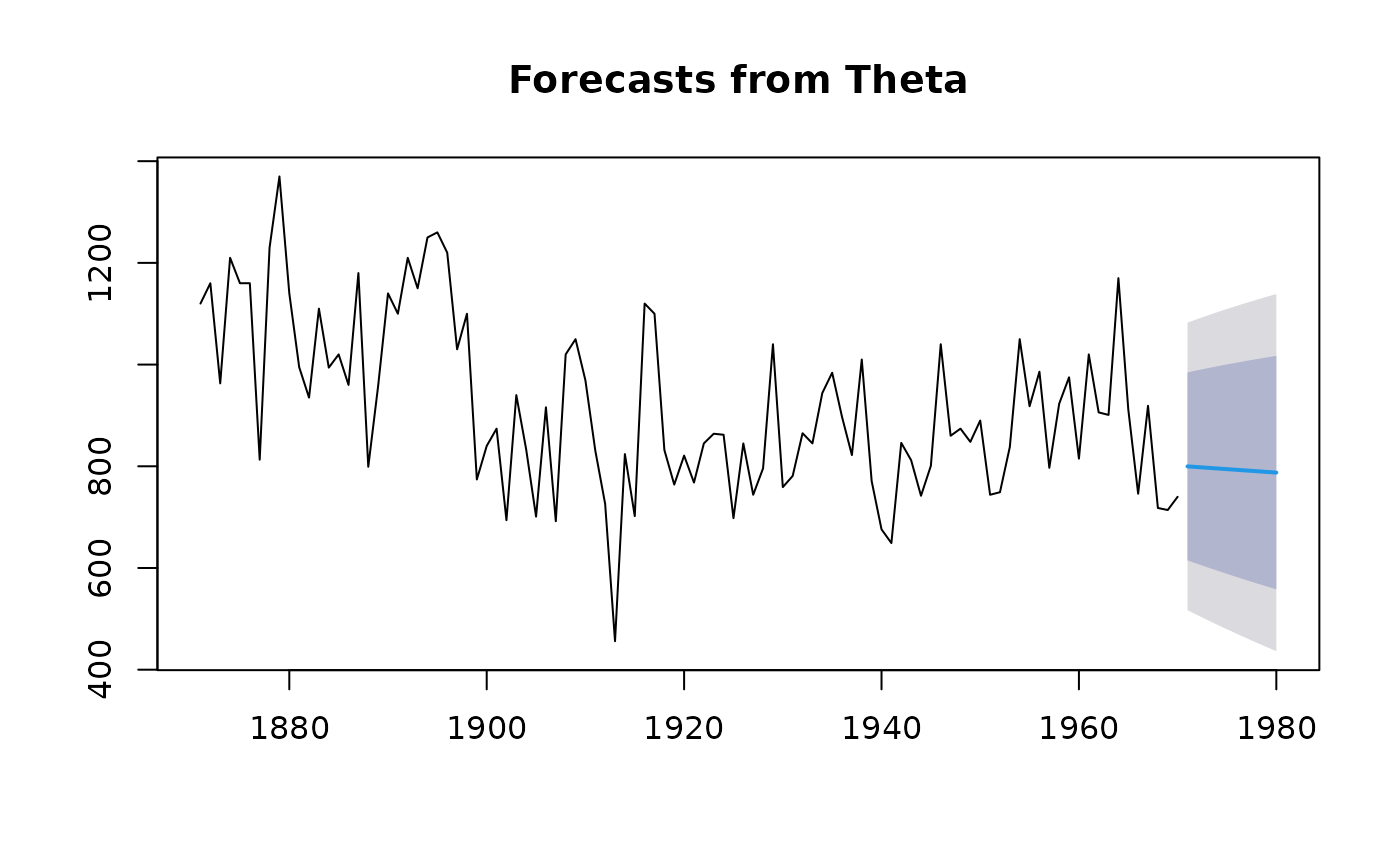Returns forecasts and prediction intervals for a theta method forecast.

thetaf(
y,
h = ifelse(frequency(y) > 1, 2 * frequency(y), 10),
level = c(80, 95),
fan = FALSE,
x = y
)

## Arguments

y

a numeric vector or time series of class ts

h

Number of periods for forecasting

level

Confidence levels for prediction intervals.

fan

If TRUE, level is set to seq(51,99,by=3). This is suitable for fan plots.

x

Deprecated. Included for backwards compatibility.

## Value

An object of class "forecast".

The function summary is used to obtain and print a summary of the results, while the function plot produces a plot of the forecasts and prediction intervals.

The generic accessor functions fitted.values and residuals

extract useful features of the value returned by rwf.

An object of class "forecast" is a list containing at least the following elements:

model

A list containing information about the fitted model

method

The name of the forecasting method as a character string

mean

Point forecasts as a time series

lower

Lower limits for prediction intervals

upper

Upper limits for prediction intervals

level

The confidence values associated with the prediction intervals

x

The original time series (either object itself or the time series used to create the model stored as object).

residuals

Residuals from the fitted model. That is x minus fitted values.

fitted

Fitted values (one-step forecasts)

## Details

The theta method of Assimakopoulos and Nikolopoulos (2000) is equivalent to simple exponential smoothing with drift. This is demonstrated in Hyndman and Billah (2003).

The series is tested for seasonality using the test outlined in A&N. If deemed seasonal, the series is seasonally adjusted using a classical multiplicative decomposition before applying the theta method. The resulting forecasts are then reseasonalized.

Prediction intervals are computed using the underlying state space model.

More general theta methods are available in the forecTheta package.

arima, meanf, rwf, ses
nile.fcast <- thetaf(Nile)